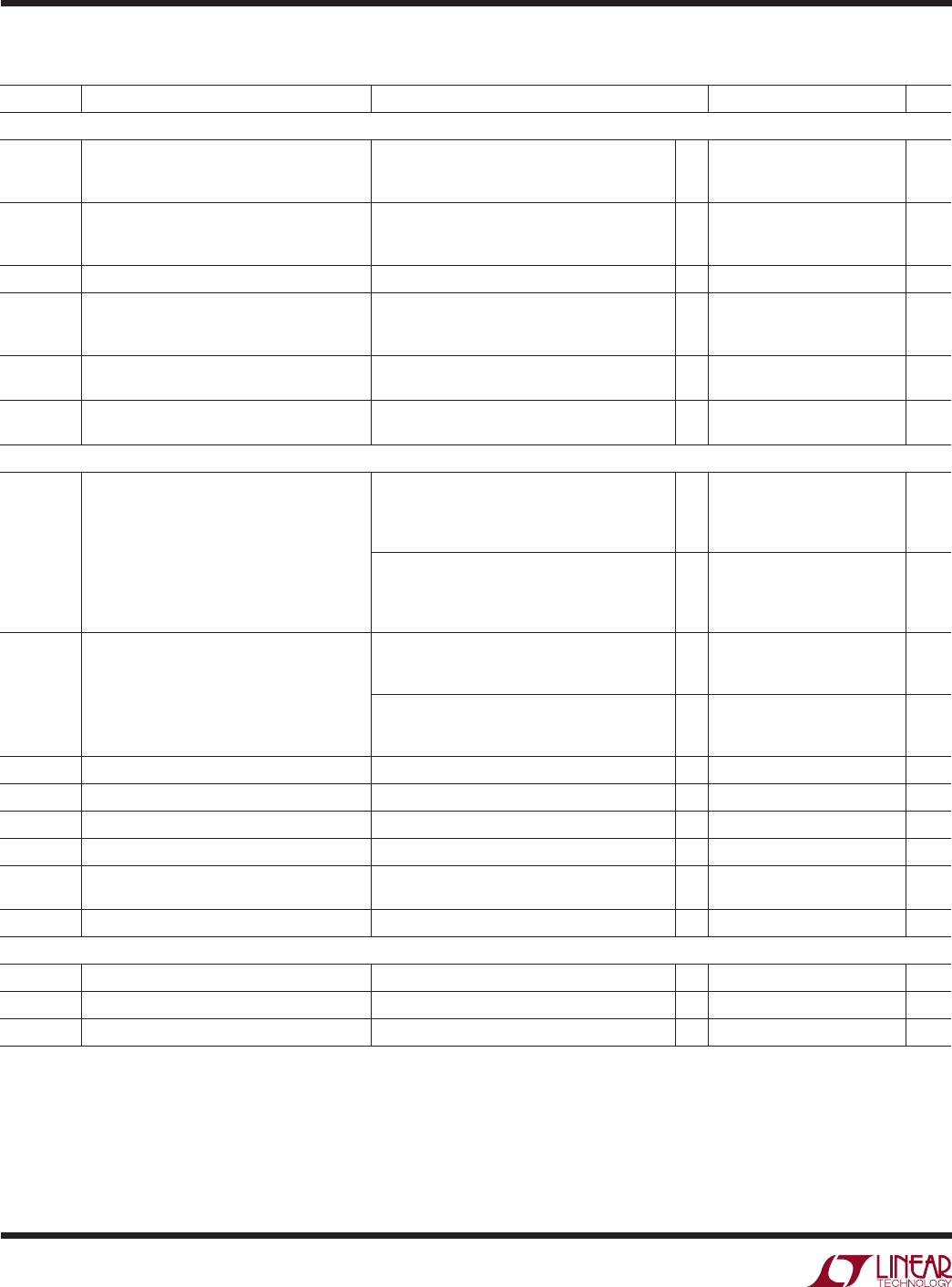# DatasheetLTC2850/LTC2851/LTC2852
4
285012fd
elecTrical characTerisTics
The l denotes the specifications which apply over the full operating
temperature range, otherwise specifications are at T
A
= 25°C. V
CC
= 3.3V, unless otherwise noted. (Note 2)
SYMBOL PARAMETER CONDITIONS MIN TYP MAX UNITS
Driver
|V
OD
| Differential Driver Output Voltage R = ∞, V
CC
= 3V (Figure 1)
R = 27Ω, V
CC
= 3V (Figure 1)
R = 50Ω, V
CC
= 3.13V (Figure 1)
l
l
l
1.5
2
V
CC
V
CC
V
CC
V
V
V
Δ|V
OD
|
Difference in Magnitude of Driver Differential
Output Voltage for Complementary Output
States
R = 27Ω or 50Ω (Figure 1)
l
0.2 V
V
OC
Driver Common Mode Output Voltage
R = 27Ω or 50Ω (Figure 1)
l
3 V
Δ|V
OC
|
Difference in Magnitude of Driver Common
Mode Output Voltage for Complementary
Output States
R = 27Ω or 50Ω (Figure 1)
l
0.2 V
I
OZD
Driver Three-State (High Impedance) Output
Current on Y and Z
DE = 0V, (Y or Z) = –7V, 12V (LTC2852)
l
±10 µA
I
OSD
Maximum Driver Short-Circuit Current –7V ≤ (Y or Z) ≤ 12V (Figure 2)
l
–250
±180 ±250
300
mA
mA
I
IN
Receiver Input Current (A, B) DE = TE = 0V, V
CC
= 0V or 3.3V, V
IN
= 12V
DE = TE = 0V, V
CC
= 0V or 3.3V, V
IN
= –7V,
l
l
–100
125 µA
µA
DE = TE = 0V, V
CC
= 0V or 3.3V, V
IN
= 12V
DE = TE = 0V, V
CC
= 0V or 3.3V, V
IN
= –7V,
l
l
–145
250 µA
µA
R
IN
Receiver Input Resistance RE = V
CC
or 0V, DE = TE = 0V,
V
IN
= –7V, –3V, 3V, 7V, 12V (Figure 3)
l
96 125
kΩ
RE = V
CC
or 0V, DE = TE = 0V,
V
IN
= –7V, –3V, 3V, 7V, 12V (Figure 3)
l
48 125
kΩ
V
TH
Receiver Differential Input Threshold Voltage –7V ≤ B ≤ 12V
l
±0.2 V
ΔV
TH
Receiver Input Hysteresis B = 0V 25 mV
V
OH
Receiver Output High Voltage I(RO) = –4mA, A-B = 200mV, V
CC
= 3V
l
2.4 V
V
OL
Receiver Output Low Voltage I(RO) = 4mA, A-B = –200mV, V
CC
= 3V
l
0.4 V
I
OZR
Output Current on RO
RE = V
CC
, 0V ≤ RO ≤ V
CC
(LTC2850, LTC2852)
l
±1 µA
I
OSR
Receiver Short-Circuit Current 0V ≤ RO ≤ V
CC
l
±85 mA
Logic
V
IH
Logic Input High Voltage V
CC
= 3.6V
l
2 V
V
IL
Logic Input Low Voltage V
CC
= 3V
l
0.8 V
I
INL
Logic Input Current
l
0 ±10 µA